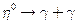Chapter 33, Problem 26PE

Chapter
Section
Textbook Problem

One decay mode for the eta−zero meson is(a) Find the energy released.(b) What is the uncertainty in the energy due to the short lifetime?(c) Write the decay in terms at the constituent quarks.(d) Verify that baryon number, lepton numbers, and charge are conserved.

To determine

(a)

The energy released in the decay of η0 particle given by η0γ+γ

Explanation

Given info:

The primary decay mode of negative pion is given by, η0γ+γ

Formula used:

Energy released = Rest energy of initial particle − sum of energies of decay products

Calculation:

In this problem the η0 particle decays by η0γ+γ

Rest energy of η0 particle =547

To determine

(b)

The uncertainty in the energy released in the decay of η0 due to its short life time.

To determine

(c)

To write the equation η0γ+γ in terms of quark structure.

To determine

(d)

The conservation of charge, baryon number and lepton numbers for the reaction η0γ+γ.

Still sussing out bartleby?

Check out a sample textbook solution.

See a sample solution

The Solution to Your Study Problems

Bartleby provides explanations to thousands of textbook problems written by our experts, many with advanced degrees!

Get Started

Find more solutions based on key concepts